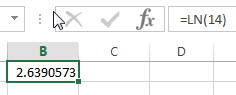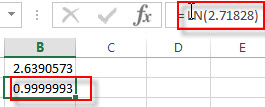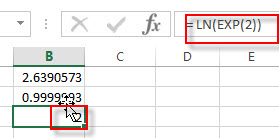## Excel LN Function

This post will guide you how to use Excel LN function with syntax and examples in Microsoft excel.

### Description

The Excel LN function returns the natural logarithm of a given number.  And the natural logarithms are based on the constant e (the value is equal to 2.718281828…).

The LN function is a build-in function in Microsoft Excel and it is categorized as a Math and Trigonometry Function.

The LN function is available in Excel 2016, Excel 2013, Excel 2010, Excel 2007, Excel 2003, Excel XP, Excel 2000, Excel 2011 for Mac.

### Syntax

The syntax of the LN function is as below:

`= LN (number)`

Where the LN function arguments is:

• number – This is a required argument. The number that you want the logarithm. And the number must be a positive real number.

Note:

• If either the supplied number argument is negative or zero, the LN function will return #NUM! Error.
• If the number argument is non-numeric, the LN function will return #VALUE! Error.
• LN is the inverse of the Exp function.

### Excel LN Function Examples

The below examples will show you how to use Excel LN Function to calculate the natural logarithm of a given number.

1# to get the natural logarithm of 14, enter the following formula in Cell B1.

`= LN (14)`2# to get the natural logarithm of the value of the constant e (2.71828), enter the following formula in Cell B2.

`= LN (2.71828)`3# to get the natural logarithm of e raised to the power 2, enter the following formula in Cell B2.

`= LN (EXP(2))`### Related Functions

• Excel LOG10 function
The Excel LOG10 function returns the base-10 logarithm of a given number.The syntax of the LOG10 function is as below:= LOG10 (number)…
• Excel LOG function
The Excel LOG function returns the logarithm of a given number based on a supplied base. And the default base argument is 10.The syntax of the LOG function is as below:= LOG (number,[base])…